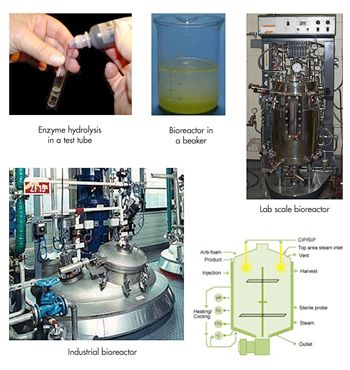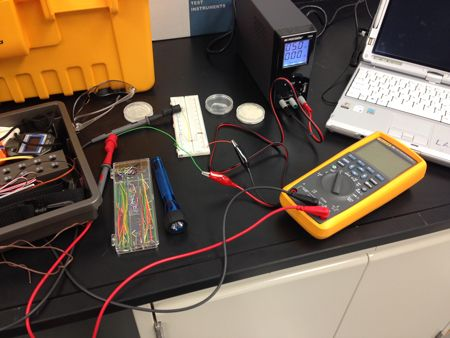Technical Articles

# Teaching Instrumentation of Biological Systems with MATLAB in a Spiral Engineering Curriculum

By P. Kumar Mallikarjunan, Virginia Tech

The Department of Biological Systems Engineering (BSE) at Virginia Tech received a National Science Foundation grant in 2004 to develop a curriculum around spiral theme-based learning. In a spiral curriculum, students repeatedly return to the same basic concepts as they solve problems of increasing complexity. We use this approach to teach not only biological systems engineering concepts but also modern engineering tools, including MATLAB®.

MATLAB is key to the spiral curriculum at Virginia Tech because it supports both the basic data analysis required for first-year projects and the data acquisition and more sophisticated statistical analyses required in later years. By the time the students have completed the fourth year of the spiral curriculum, they have used MATLAB to analyze and understand progressively more complex bioprocesses, first in a test tube, then in a beaker, in pilot-scale reactors in the lab, and finally, in industrial processing facilities (Figure 1).Figure 1. Unit progression in the biological systems spiral curriculum.

## Implementing a Spiral Curriculum with MATLAB

One goal of a spiral curriculum is to prepare students to start practicing their profession immediately after graduation. To reach this goal, we knew that we had to engage the students in solving practical, authentic biological systems engineering problems from day one.

In their first year, BSE students take General Chemistry and Introduction to Engineering. At this introductory level they study a simple bioprocess in which they add an enzyme to a test tube containing a substrate. The enzyme catalyzes a hydrolysis reaction that changes the color of the substrate from blue to yellow. The students monitor and measure this change using a spectrophotometer, and perform a linear regression of the measured data in MATLAB and estimate the coefficients in a Micheles-Menton equation. After seeing firsthand how an enzyme works, they learn how enzymes are used in chemical engineering, bioremediation, and real-world bioreactor processes.

In the second-year course Introduction to Biological Systems Engineering, assignments are more advanced. For example, instead of simply adding an enzyme to a substrate in a beaker, the students use a microorganism that produces the enzyme; and instead of simply measuring a color change, they extract material from the bioprocess, categorize it, and use MATLAB to analyze the material balance and energy balance of the process.

In the third-year course Unit Operations in BSE, students complete projects comparable in scale and scope to lab experiments conducted by practicing biological systems engineers. Using a pilot-scale continuous distiller reactor, students design and conduct unit operation experiments in which they control the dissolved oxygen and pH of the reactor and use MATLAB to analyze and interpret the test results. Third-year students also take a numerical analysis course in which they further develop their skills for solving differential equations in MATLAB.

In their final year, students complete courses on bioprocess engineering, industrial bioprocessing, and bioprocessing plant design. The emphasis is on learning how to design a complete reactor. As part of their studies, the students visit a working processing facility in which the reactor is several stories tall.

## Teaching Bioprocess Instrumentation with MATLAB

Before tackling their bioreactor design projects, fourth-year students learn instrumentation and control using MATLAB, Data Acquisition Toolbox™, and Instrument Control Toolbox™. Instrumentation, Measurement, and Control in Biological Systems used to be taught by faculty from the engineering science and mechanics department. Because the course included engineering students from other departments, the lab assignments were usually not relevant to bioprocess engineering. Another drawback was that the students had to learn a new graphical programming environment just for that course, and class time had to be set aside to teach them how to use it. My BSE colleagues and I decided to redesign the course and teach it ourselves. We developed lab assignments specific to bioengineering, and changed the required software to MATLAB, Data Acquisition Toolbox, and Instrument Control Toolbox.

We applied the spiral concept to labs in the course, progressing from assignments in which the students simply import measured data into MATLAB to assignments that require more advanced data acquisition techniques. In the first lab the students measure voltages and resistances with a multimeter, recording the data in a spreadsheet. They import the data into MATLAB, generate a scatter plot, and perform linear regression. For the next two labs, students take voltage measurements using a thermocouple, thermistor, and optical sensor (Figure 2).Figure 2. Instrumentation lab setup.

They use a USB connection to import the multimeter data directly into MATLAB for analysis. After an assignment on performing a discrete fast Fourier transform using MATLAB, they continue with a lab in which they use RS232C and GPIB within Data Acquisition Toolbox to acquire colorimeter and oscilloscope data. Subsequent labs cover chromatography, pressure measurement, flow measurement, force measurement using a strain gauge, and biogas pressure monitoring using a manometer. For measuring pressure, flow, and force, the students connect the sensors using a data acquisition board and Instrument Control Toolbox from MATLAB.

For the final project, each student submits an experimental design, and the class selects one of the designs to complete together. One recent project involved producing biogas from manure and analyzing thermocouple and pressure sensor data in MATLAB to monitor the process and maximize output. Another involved using biopolymers as packaging to extend the shelf life of bananas. For this project, students measured color, texture, pH, and puncture force, processing the data using statistical tools in MATLAB and using the results to gauge the bananas’ ripeness.

Student surveys given before and after the course underscored the value not only of this project, but of the course overall. The number of students who strongly agreed that they understood the importance of controlling biological systems to maximize results rose from 3 to 17. The students also reported significant improvement in their understanding of sensors and controls, as well as increased confidence in their ability to use MATLAB to connect to hardware for data collection and then to import, plot, and curve fit the collected data. The number of students who agreed that the MATLAB skills they learned would have broader use in future courses and careers almost tripled.